# ISEE Middle Level Math : How to find the area of a rectangle

## Example Questions

### Example Question #71 : Quadrilaterals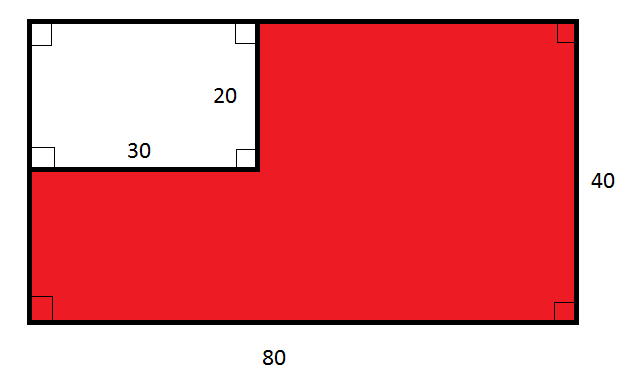Note: Figure NOT drawn to scale.

Refer to the above diagram. Give the ratio of the area of the red region to that of the white region.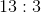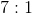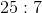The correct answer is not given among the other choices.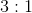Explanation:

The large rectangle has length 80 and width 40, and, consequently, area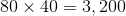.

The white region is a rectangle with length 30 and width 20, and, consequently, area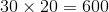.

The red region, therefore, has area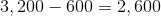The ratio of the area of the red region to that of the white region is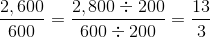That is, 13 to 3.

### Example Question #78 : Quadrilaterals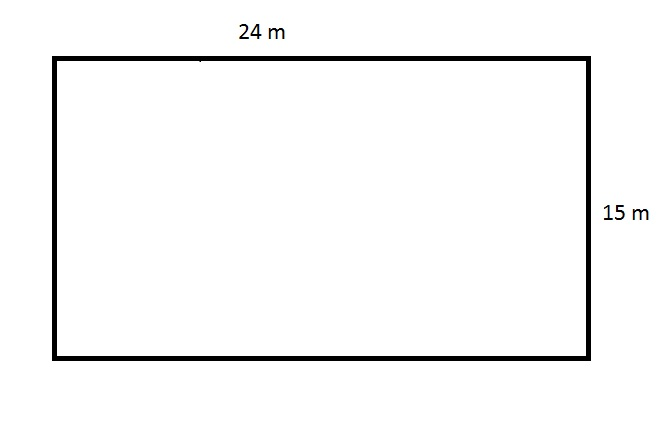The above figure depicts the rectangular swimming pool at an apartment. The apartment manager needs to purchase a tarp that will cover this pool completely, but the store will only sell the material in multiples of one hundred square meters. How many square meters will the manager need to buy?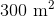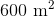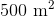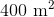Insufficient information is given to answer the question.Explanation:

The tarp needed to cover this pool must be, at minimum, the product of its length and width, or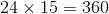square meters.

The manager will need to buy a number of square yards of tarp equal to the next highest multiple of one hundred, which is 400 square meters.

### Example Question #2001 : Isee Middle Level (Grades 7 8) Mathematics Achievement

The four angles of a square are labeled A, B, C, and D. What is the sum of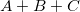?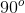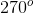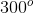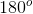Explanation:

In a square, each angle is 90 degrees.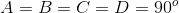We can plug in 90 for each variable and find the sum.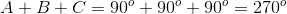### Example Question #81 : Quadrilaterals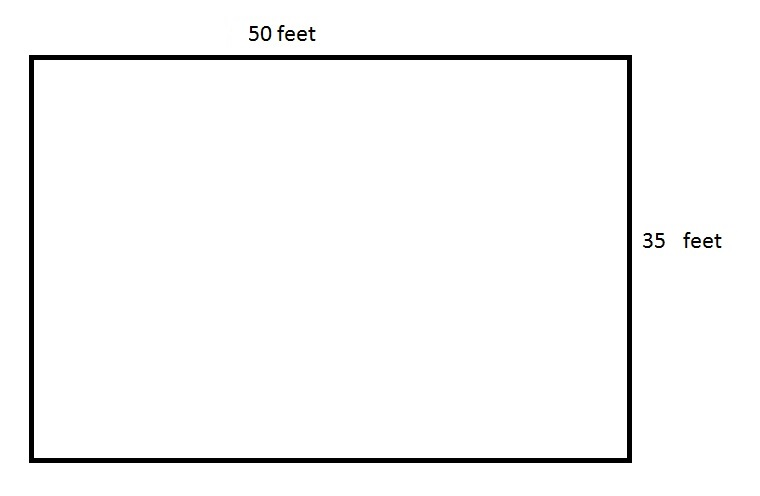The above depicts a rectangular swimming pool for an apartment. The pool is six feet deep everywhere.

An apartment manager wants to paint the four sides and the bottom of the swimming pool. How many square feet will he need to paint?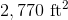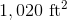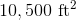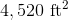The correct answer is not given among the other responses.Explanation:

The bottom of the swimming pool has area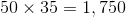square feet.

There are two sides whose area is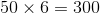square feet,

and two sides whose area is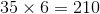square feet.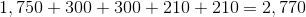square feet.

### Example Question #21 : How To Find The Area Of A Rectangle

If the angles of a quadrilateral are equal to,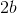,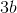, and, what is the value of?Explanation:

Given that there are 360 degrees in a quadrilateral,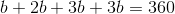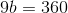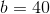### Example Question #161 : Geometry

What is the value of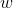if the angles of a quadrilateral are equal todegrees,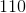degrees,degrees, and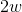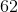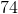Explanation:

Given that there are 360 degrees in a quadrilateral,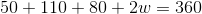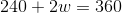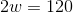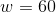### Example Question #84 : Quadrilaterals

If the length of a rectangle is 7.5 feet and the width is 2 feet, what is the value ofif the area is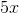?Explanation:

The area of a rectangle is calculated by multiplying the length by the width. Here, the length is 7.5 and the width is 2, so the area will be 15.

Given that the area is also equal to, the value ofwill be 3, given that 3 times 5 is 15.

### Example Question #91 : Quadrilaterals

Which of the following is equal to the area of a rectangle with length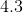meters and width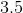meters?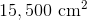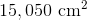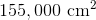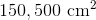Explanation:

Multiply each dimension byto convert meters to centimeters: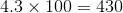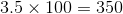Multiply these dimensions to get the area of the rectangle in square centimeters: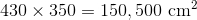### Example Question #21 : Rectangles

Find the area of a rectangle whose length is 6 and width is 5.Explanation:

To solve, simply use the formula for the area of a rectangle.

In this particular case the length and width are given,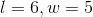.

Thus: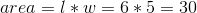### Example Question #91 : Quadrilaterals

The area of a four-sided room that has dimensions of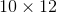will be the four wall lengths all added to together.  True or False?

True

False